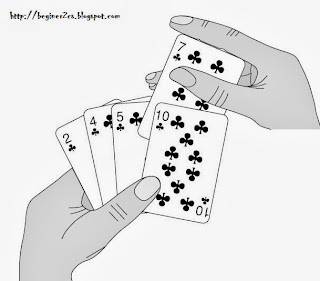## Tuesday, 22 October 2013

### Insertion Sort (concept & algorithm)insertion like cards

An array A with N elements A, A … A[N] is in memory

Insertion Sort scan A from A to A[N] inserting each elements A[k] into its proper position in the previously sorted subarray A, A, …. A[K-1]

Pass 1: A by itself is trivially sorted
Pass 2: A is inserted either before or after A so that A, A is sorted
Pass 3: A is inserted in its proper place in A, A, that is before A, between A and A or after A so that A, A, A is sorted

Pass 4: A is inserted in its proper place in A, A, A so that A, A, A, A  is sorted.
.
.
Pass N: A[N] is inserted in its proper place in A, A, A, .. A[N-1]  so that A, A, A, A ,  A[N]  is sorted.

### Insertion Sort Example :

Sort an array A with 8 elements
77, 33, 44, 11, 88, 66, 55

#### Insertion Algorithm :

This algorithm sort an array with N elements
 Set A = -∞ [Initialize a delimiter]
 Repeat Steps 3 to 5 for K = 2, 3, …, N
 Set TEMP = A[K] and PTR = K-1
 Repeat while TEMP < A[PTR]
(a) Set A[PTR+1] = A[PTR]
(b) Set PTR = PTR -1
 Set A[PTR+1] = TEMP
 Exit

Related Post : TOWER OF HANOI

#### Complexity of Insertion Sort :

Worst Case: O(n^2)

Average Case: O(n^2)

### C++ program :

1. /*
2. Insertion sort   //most optimise algorithm
3. beginer2cs.blogspot.com
4. */
5. #include<iostream>
6. #include<malloc.h>
7. using namespace std;
8. main()
9. {
10.         int *arr,n,i,j,key;
11.
12.         cout<<"Number of element to sort : ";
13.         cin>>n;
14.
15.         arr=(int*)malloc(sizeof(int)*n); //allocating space to array
16.
17.         cout<<"Enter element of array \n";
18.         for(i=0;i<n;i++)
19.           cin>>*(arr+i);  //taking input
20.
21.         /********** insertion sort begin  ************/
22.         for(j=1;j<n;j++)  //start from second element
23.           {
24.                 key=*(arr+j); //storing j'th element to temp variable
25.                 i=j-1;
26.                 while(i>=0 && key<*(arr+i))
27.                 {
28.                         *(arr+i+1)=*(arr+i);
29.                         i--;
30.                 }
31.                 *(arr+i+1)=key ;  //final position where key placed
32.           }
33.         /***************sorting complete ********************/
34.
35.         //Print sorted array
36.         cout<<"sorted list \n ";
37.         for(i=0;i<n;i++)
38.          cout<<*(arr+i)<<"  ";
39.
40.          return 0;
41. }